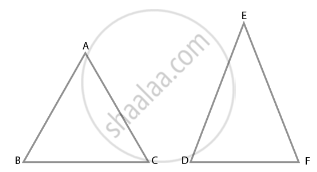# If ΔABC ~ ΔEDF and ΔABC is not similar to ΔDEF, then which of the following is not true? - Mathematics

MCQ

If ΔABC ~ ΔEDF and ΔABC is not similar to ΔDEF, then which of the following is not true?

#### Options

• BC . EF = A C. FD

• AB . EF = AC . DE

• BC . DE = AB . EF

• BC . DE = AB . FD

#### Solution

BC . DE = AB . EF

Explanation:

We know that, If sides of one triangle are proportional to the side of the other triangle, and the corresponding angles are also equal, then the triangles are similar by SSS similarity.So, ∆ABC ∼ ∆EDF

Using similarity property,

(AB)/(ED) = (BC)/(DF) = (AC)/(EF)

Taking (AB)/(ED) = (BC)/(DF), we get

(AB)/(ED) = (BC)/(DF)

AB.DF = ED.BC

So, option (d) BC · DE = AB · FD is true

Taking (BC)/(DF) = (AC)/(EF), we get

(BC)/(DF) = (AC)/(EF)

⇒ BC.EF = AC.DF

So, option (a) BC · EF = AC · FD is true

Taking (AB)/(ED) = (AC)/(EF), we get,

(AB)/(ED) = (AC)/(EF)

AB.EF = ED.AC

So, option (b) AB · EF = AC · DE is true.

Concept: Similarity of Triangles
Is there an error in this question or solution?

#### APPEARS IN

NCERT Mathematics Exemplar Class 10
Chapter 6 Triangles
Exercise 6.1 | Q 3 | Page 61
Share